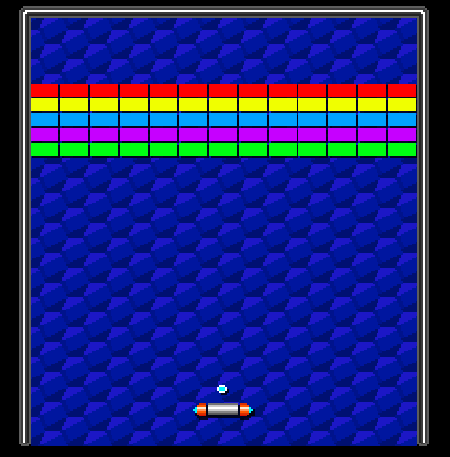# 【Unity3D开发小游戏】打砖块游戏

## 三、教程

### 4、添加边界选择图片另存为，把资源导入到项目中 Sprite文件夹中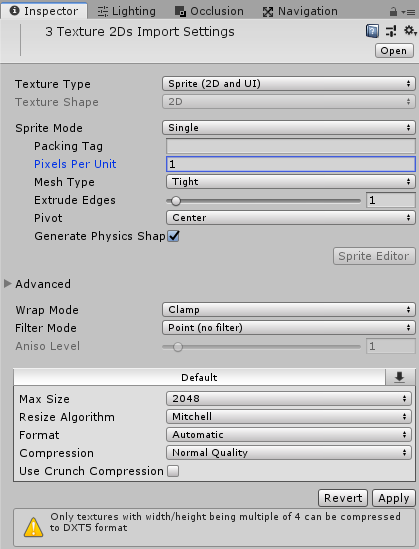然后把这些边界摆放到场景中：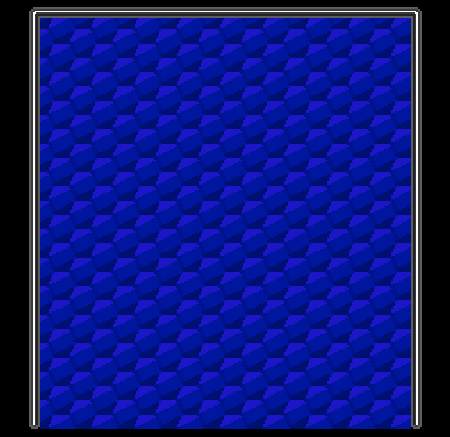添加边界的碰撞： 选中边界：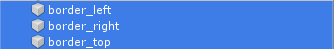添加碰撞器: Add Component -> Physics 2D -> Box Collider 2D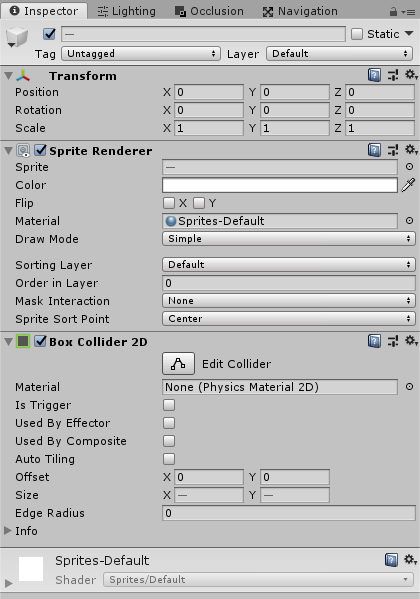### 6、球拍移动

``````using UnityEngine;
using System.Collections;

public class Racket : MonoBehaviour {

// Use this for initialization
void Start () {

}

// Update is called once per frame
void Update () {

}
}
``````

``````using UnityEngine;
using System.Collections;

public class Racket : MonoBehaviour {

void FixedUpdate () {

}
}
``````

``````using UnityEngine;
using System.Collections;

public class Racket : MonoBehaviour {
// Movement Speed
public float speed = 150;

void FixedUpdate () {

}
}
``````

``````void FixedUpdate () {
// Get Horizontal Input
float h = Input.GetAxisRaw("Horizontal");
}
``````

``````void FixedUpdate () {
// Get Horizontal Input
float h = Input.GetAxisRaw("Horizontal");

// Set Velocity (movement direction * speed)
GetComponent<Rigidbody2D>().velocity = Vector2.right * h * speed;
}
``````

### 8、添加球的脚本

``````using UnityEngine;
using System.Collections;

public class Ball : MonoBehaviour {

// Use this for initialization
void Start () {

}

// Update is called once per frame
void Update () {

}
}
``````

``````using UnityEngine;
using System.Collections;

public class Ball : MonoBehaviour {

// Use this for initialization
void Start () {

}
}
``````

``````using UnityEngine;
using System.Collections;

public class Ball : MonoBehaviour {
// Movement Speed
public float speed = 100.0f;

// Use this for initialization
void Start () {
GetComponent<Rigidbody2D>().velocity = Vector2.up * speed;
}
}
``````

### 9、球拍碰撞角``````using UnityEngine;
using System.Collections;

public class Ball : MonoBehaviour {
// Movement Speed
public float speed = 100.0f;

// Use this for initialization
void Start () {
GetComponent<Rigidbody2D>().velocity = Vector2.up * speed;
}

void OnCollisionEnter2D(Collision2D col) {
// This function is called whenever the ball
// collides with something
}
}
``````

y值永远是1，因为我们希望它飞向顶部，-1是飞向底部。x值是它击中球拍的位置，也就是它的速度。

``````1  -0.5  0  0.5   1  <- x值取决于它被击中的位置
===================  <- 这是那个球拍
``````

``````float hitFactor(Vector2 ballPos, Vector2 racketPos,
float racketWidth) {
// ascii art:
//
// 1  -0.5  0  0.5   1  <- x value
// ===================  <- racket
//
return (ballPos.x - racketPos.x) / racketWidth;
}
``````

``````void OnCollisionEnter2D(Collision2D col) {
// Hit the Racket?
if (col.gameObject.name == "racket") {
// Calculate hit Factor
float x=hitFactor(transform.position,
col.transform.position,
col.collider.bounds.size.x);

// Calculate direction, set length to 1
Vector2 dir = new Vector2(x, 1).normalized;

// Set Velocity with dir * speed
GetComponent<Rigidbody2D>().velocity = dir * speed;
}
}
``````

### 10、添加块

``````using UnityEngine;
using System.Collections;

public class Block : MonoBehaviour {

// Use this for initialization
void Start () {

}

// Update is called once per frame
void Update () {

}
}
``````

``````using UnityEngine;
using System.Collections;

public class Block : MonoBehaviour {

void OnCollisionEnter2D(Collision2D collisionInfo) {
// Destroy the whole Block
Destroy(gameObject);
}
}
``````